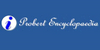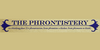### IsocostIn economics, the isocost is the set of combinations of goods that have the same total cost; this can be represented by a curve on a graph. In producer theory isocosts refer to inputs and, making certain assumptions, there will be a unique minimum cost of producing a given level of output, which can be represented as the point at which a particular...
Found on http://www.probertencyclopaedia.com/browse/JI.HTM

### Isocostwhere w represents the wage rate of labour, r represents the rental rate of capital, K is the amount of capital used, L is the amount of labour used, and C is the total cost of acquiring those quantities of the two inputs. The isocost line is combined with the isoquant map to determine the optimal production point at any given level of output. Spe...
Found on http://en.wikipedia.org/wiki/Isocost

### isocostline connecting points of equal cost
Found on http://phrontistery.info/i.html
No exact match found